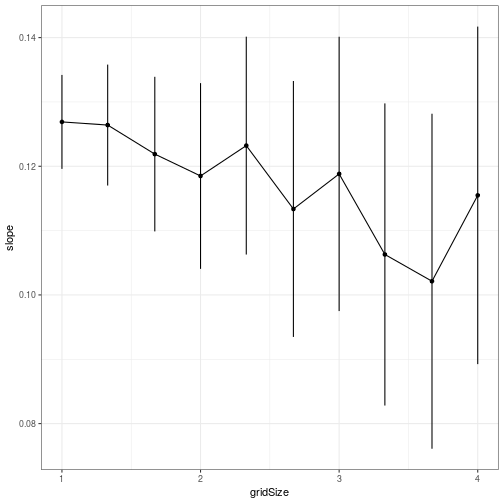## Appendix S4

Supporting information in Valcu, M., Dale, J., and Kempenaers, B. (2012). rangeMapper: a platform for the study of macroecology of life-history traits. Global Ecology and Biogeography 21, 945-951.

The example shown here is run on the wrens dataset which is part of the package. The wrens dataset has 84 species while the case study presented in the paper was run on 8434 bird species. Therefore both the settings and the results shown below are not identical with the results presented in Valcu et al 2012.

### Project Set Up

Define grid size vector of increasing grid sizes.

``````require(rangeMapper)
data(wrens) # life history data
"extdata", "wrens", "vector_combined"), "wrens", verbose = FALSE)

gridSizes = round(seq(from = 1, to = 4, length.out = 10), 2)
``````

### Run `log10(median_body_mass) ~ sqrt(species_richness)` regression for each grid size

``````output = list()

for( i in 1:length(gridSizes) ) {

d = subset(wrens, select = c('sci_name', 'body_mass') )
con = ramp("wrens.sqlite", gridSize = gridSizes[i], spdf = breding_ranges,
biotab = d, ID = "sci_name",
FUN = "median", overwrite = TRUE)
o = rangeMap.fetch(con, spatial = FALSE)

output[[i]] = lm(log10(median_body_mass) ~ sqrt(species_richness), data= o)

}
``````

### Extract and plot regression parameters

``````X = lapply(output,
function(x) data.frame(slope = coef(x),
ciu = confint(x)[2,1],
cil = confint(x)[2,2])
)
X = do.call(rbind, X)
X\$gridSize = gridSizes

require(ggplot2)

ggplot(X, aes(x = gridSize, y = slope)) +
geom_errorbar(aes(ymin = cil, ymax = ciu), width= 0) +
geom_line() +
geom_point() +
theme_bw()
``````